NCERT Solutions for Class 10 Maths Chapter 8 Introduction to Trigonometry Ex 8.1 are part of NCERT Solutions for Class 10 Maths. Here we have given NCERT Solutions for Class 10 Maths Chapter 8 Introduction to Trigonometry Ex 8.1.

 Board CBSE Textbook NCERT Class Class 10 Subject Maths Chapter Chapter 8 Chapter Name Introduction to Trigonometry Exercise Ex 8.1 Number of Questions Solved 11 Category NCERT Solutions

NCERT Solutions for Class 10 Maths Chapter 8 Introduction to Trigonometry Ex 8.1

Exercise 8.1 Class 10 Question 1.
In ∆ABC right angled at B, AB = 24 cm, BC = 7 cm. Determine:
(i) sin A, cos A
(ii) sin C, cos C
Solution: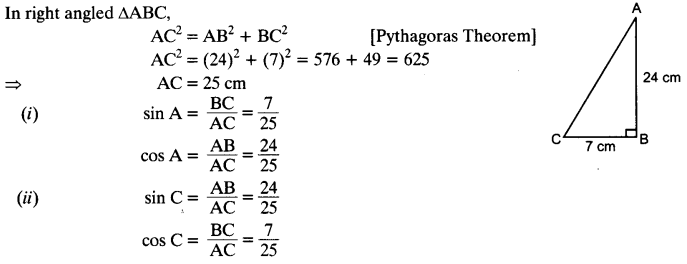Class 10 Ex 8.1 Question 2.
In given figure, find tan P – cot R.
Solution: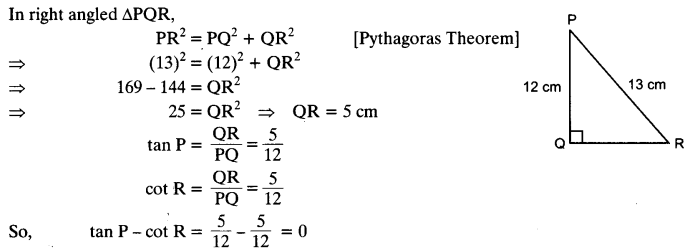Trigonometry Class 10 Question 3.
If sin A = $$\frac { 3 }{ 4 }$$ , calculate cos A and tan A.
Solution: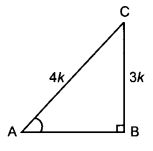sin A = $$\frac { 3 }{ 4 }$$
sin A = $$\frac { BC }{ AC }$$ = sin A = $$\frac { 3 }{ 4 }$$
Let BC = 3k and AC = 4k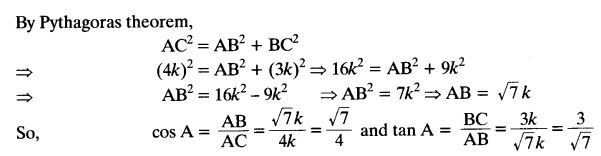Ex 8.1 Class 10 Question 4.
Given 15 cot A = 8, find sin A and sec A.
Solution:Class 10 Trigonometry Ex 8.1 Question 5.
Given sec θ = $$\frac { 13 }{ 12 }$$ , calculate all other trigonometric ratios.
Solution: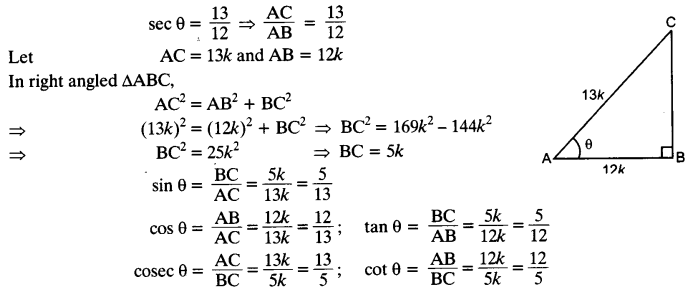Ex 8.1 Class 10 Question 6.
If ∠A and ∠B are acute angles such that cos A = cos B, then show that ∠A = ∠B.
Solution: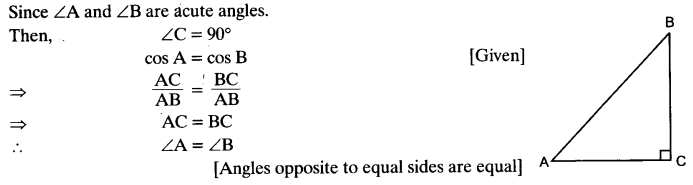Ex 8.1 Class 10 Question 7.
If cot θ = $$\frac { 7 }{ 8 }$$, evaluate:
(i) $$\frac { \left( 1+sin\theta \right) \left( 1-sin\theta \right) }{ \left( 1+cos\theta \right) \left( 1-cos\theta \right)}$$
(ii) cot²θ
Solution: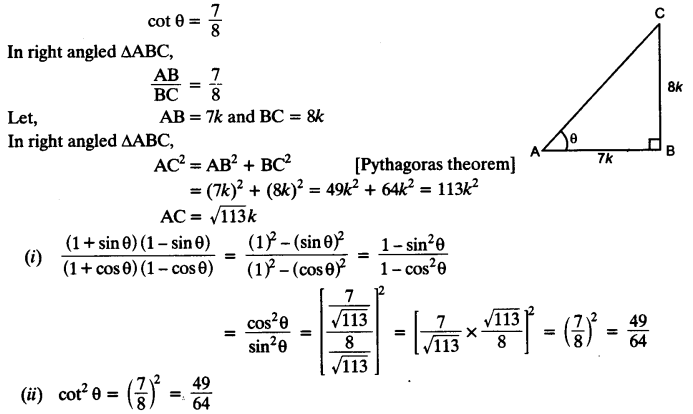Trigonometry NCERT Class 10 Ex 8.1 Question 8.
If 3 cot A = 4, check whether $$\frac { 1-tan^{ 2 }A }{ 1+tan^{ 2 }A }$$ = cos² A – sin² A or not.
Solution: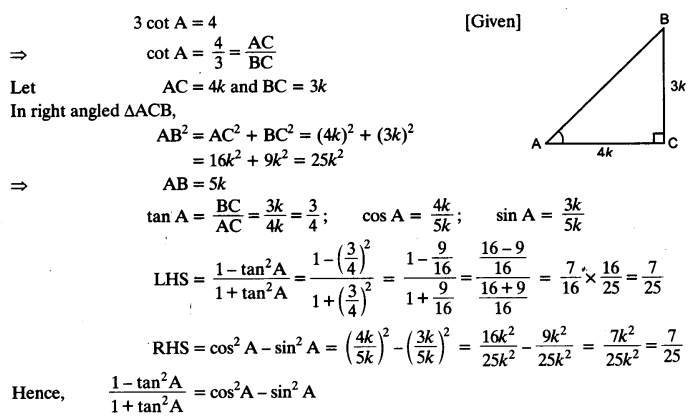Ex 8.1 Class 10 Maths Solutions Question 9.
In triangle ABC, right angled at B, if tan A = $$\frac { 1 }{ \surd 3 }$$, find the value of:
(i) sin A cos C + cos A sin C
(ii) cos A cos C – sin A sin C
Solution:Class 10 Ex 8.1 Question 10.
In ΔPQR, right-angled at Q, PR + QR = 25 cm and PQ = 5 cm. Determine the values of sin P, cos P and tan P.
Solution: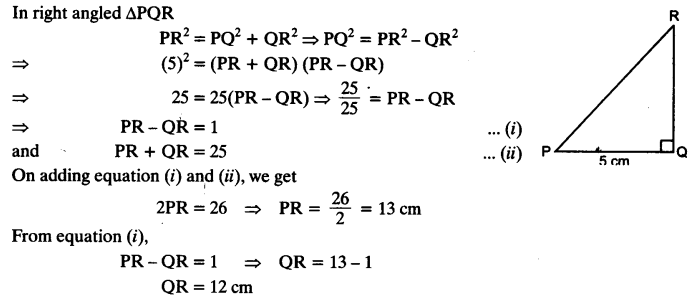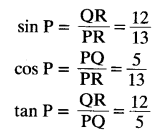NCERT Solutions For Class 10 Maths Chapter 8 Question 11.
State whether the following statements are true or false. Justify your answer.
(i) The value of tan A is always less than 1.
(ii) sec A = $$\frac { 12 }{ 5 }$$ for some value of angle A.
(iii) cos A is the abbreviation used for the cosecant of angle A.
(iv) cot A is the product of cot and A.
(v) sin θ = $$\frac { 4 }{ 3 }$$ for some angle.
Solution:
(i) tan 60° = √3 , Since √3 > 1. (False)
(ii) sec A is always ≥ 1. (True)
(iii) cos A is the abbreviation for cosine A. (False)
(iv) cot without ∠A is meaningless. (False)
(v) sin θ can never be greater than 1.
∴ sin θ = $$\frac { P }{ H }$$ , hypotenuse is always greater than other two sides. (False)

We hope the NCERT Solutions for Class 10 Maths Chapter 8 Introduction to Trigonometry Ex 8.1, help you. If you have any query regarding NCERT Solutions for Class 10 Maths Chapter 8 Introduction to Trigonometry Ex 8.1, drop a comment below and we will get back to you at the earliest.